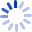Integral Geometry and Convolution Equations

Integral geometry deals with the problem of determining functions by their integrals over given families of sets. These integrals de?ne the corresponding integraltransformandoneofthemainquestionsinintegralgeometryaskswhen this transform is injective. On the other hand, when we work with complex measures or forms, operators appear whose kernels are non-trivial but which describe important classes of functions. Most of the questions arising here relate, in one way or another, to the convolution equations. Some of the well ...

Cash for Textbooks